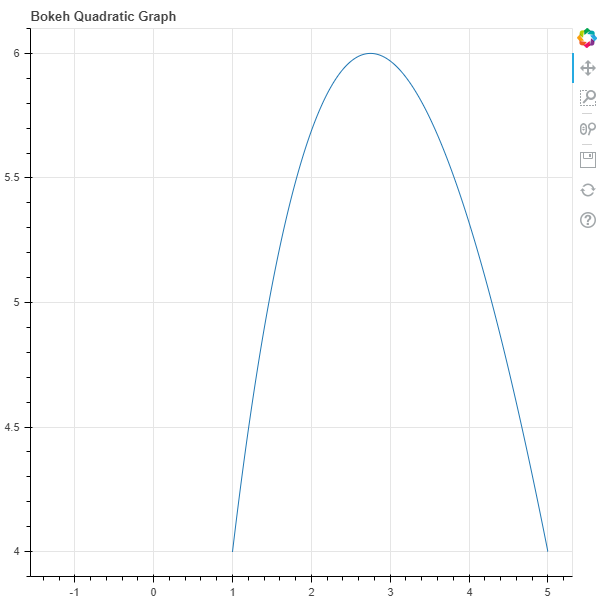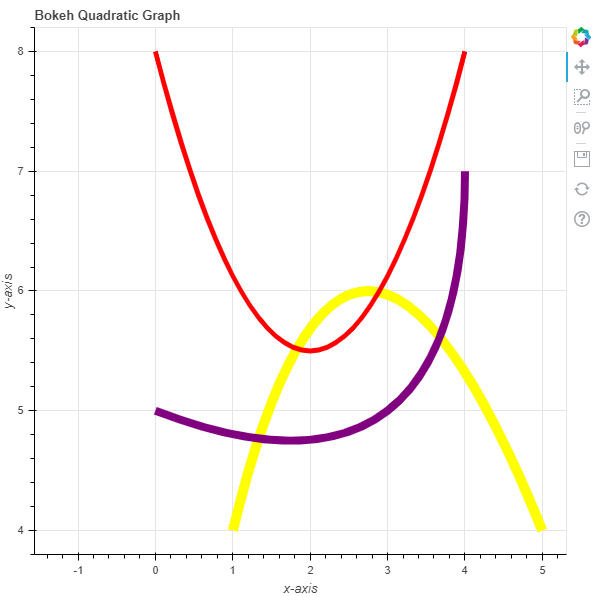Related Articles

# Python Bokeh – Plotting Quadratic Curves on a Graph

• Last Updated : 10 Jul, 2020

Bokeh is a Python interactive data visualization. It renders its plots using HTML and JavaScript. It targets modern web browsers for presentation providing elegant, concise construction of novel graphics with high-performance interactivity.

Bokeh can be used to plot quadratic curves on a graph. Plotting quadratic curves on a graph can be done using the `quadratic()` method of the `plotting` module.

Parameters :

• x0 : x-coordinates of the starting points of the quadratic
• y0 : y-coordinates of the starting points of the quadratic
• x1 : x-coordinates of the ending points of the quadratic
• y1 : y-coordinates of the ending points of the quadratic
• cx : x-coordinates of the control points of the quadratic
• cy : y-coordinates of the control points of the quadratic

Returns : an object of class `GlyphRenderer`

Example 1 : In this example we will be using the default values for plotting the graph.

 `# importing the modules``from` `bokeh.plotting ``import` `figure, output_file, show``       ` `# file to save the model``output_file(``"gfg.html"``)``       ` `# instantiating the figure object``graph ``=` `figure(title ``=` `"Bokeh Quadratic Graph"``)``     ` `# the points to be plotted``x0 ``=` `1``y0 ``=` `4``x1 ``=` `5``y1 ``=` `4``cx ``=` `2.5``cy ``=` `8`` ` `# plotting the graph``graph.quadratic(x0, y0,``                ``x1, y1,``                ``cx, cy)``     ` `# displaying the model``show(graph)`

Output :Example 2 : In this example we will be plotting multiple quadratic curves with various other parameters.

 `# importing the modules ``from` `bokeh.plotting ``import` `figure, output_file, show `` ` `# file to save the model ``output_file(``"gfg.html"``) ``      ` `# instantiating the figure object ``graph ``=` `figure(title ``=` `"Bokeh Quadratic Graph"``) `` ` `# name of the x-axis ``graph.xaxis.axis_label ``=` `"x-axis"``      ` `# name of the y-axis ``graph.yaxis.axis_label ``=` `"y-axis"`` ` `# points to be plotted``x0 ``=` `[``1``, ``0``, ``0``]``y0 ``=` `[``4``, ``8``, ``5``]``x1 ``=` `[``5``, ``4``, ``4``]``y1 ``=` `[``4``, ``8``, ``7``]``cx ``=` `[``2.5``, ``2``, ``4``]``cy ``=` `[``8``, ``3``, ``4``]`` ` `# line color value of the quadratic``line_color ``=` `[``"yellow"``, ``"red"``, ``"purple"``]`` ` `# line width value of the quadratic``line_width ``=` `[``10``, ``5``, ``8``]`` ` `# plotting the graph ``graph.quadratic(x0, y0,``                ``x1, y1,``                ``cx, cy,``                ``line_color ``=` `line_color,``                ``line_width ``=` `line_width) ``      ` `# displaying the model ``show(graph)`

Output :Attention geek! Strengthen your foundations with the Python Programming Foundation Course and learn the basics.

To begin with, your interview preparations Enhance your Data Structures concepts with the Python DS Course. And to begin with your Machine Learning Journey, join the Machine Learning – Basic Level Course

My Personal Notes arrow_drop_up